AC Generator or Dynamo

An electric generator converts mechanical energy into electrical energy. The generation of alternating currents (ac) is based on the phenomenon of electromagnetic induction. A generator has a coil wound on an armature which rotates in a magnetic field. See figure 17. A simple ac generator consists of a rectangular coil mounted on a rotor shaft. It is rotated at a constant frequency in a uniform magnetic field, B by external means. Such a generator is shown in figure17. The axis of rotation of the coil is perpendicular to the direction of the magnetic field. Because of rotation of the coil, the magnetic flux through it changes and consequently an emf is induced in the coil. The coil is connected to an external circuit by means of slip rings and brushes.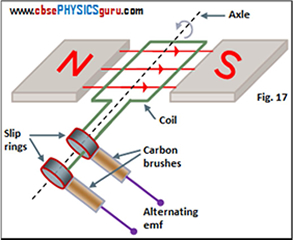If the coil rotates with an angular velocity ω and initially (at t = 0) its plane is perpendicular to the field (i.e. θ = 00), the angle θ between the magnetic field vector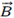and the area vector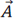of the coil at any instant t is θ = ?t. The flux linked with the coil at time t is given by Φ B = BA cos θ = BA cos ωt

If the coil has N turns, then the induced emf is given by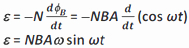This is the instantaneous value of the emf. Here NBA ω is the maximum or peak value of the emf. It can be denoted by ε0. Thus,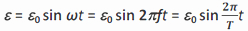where ω = 2 π f = 2 π / T and f is the frequency and T the time period of the revolution of generator coil.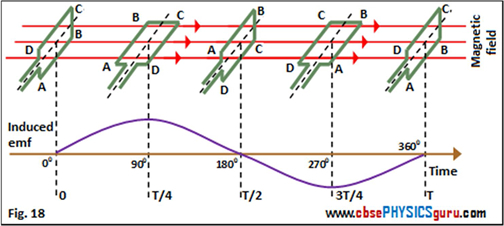Clearly, the emf has its maximum value at θ = 900 (sin 900 = + 1) or θ = 2700 (sin 2700 = - 1), as the change of flux is greatest at these points. See figure 18. It is also clear that induced emf varies sinusoidally with time. Induced emf lags behind the flux by π / 2 in phase. It means that when the flux is maximum (i.e., the plane of the coil is perpendicular to the field), the emf is zero and when the flux is zero (i.e., the plane of the coil is parallel to the field), the emf is maximum. See figure 19.

The current so induced is called alternating current (ac) because its direction changes periodically.

In most of the commercial generators, the coils are held stationary and it is the electromagnets which are rotated. The frequency of rotation is 50 Hz in India (also the frequency of ac). In certain countries such as USA, it is 60 Hz.

CBSE Electromagnetic Induction ( With Hint / Solution)
Class XII (By Mr. Ashis Kumar Satapathy)
email - [email protected]

Electromagnetic Induction